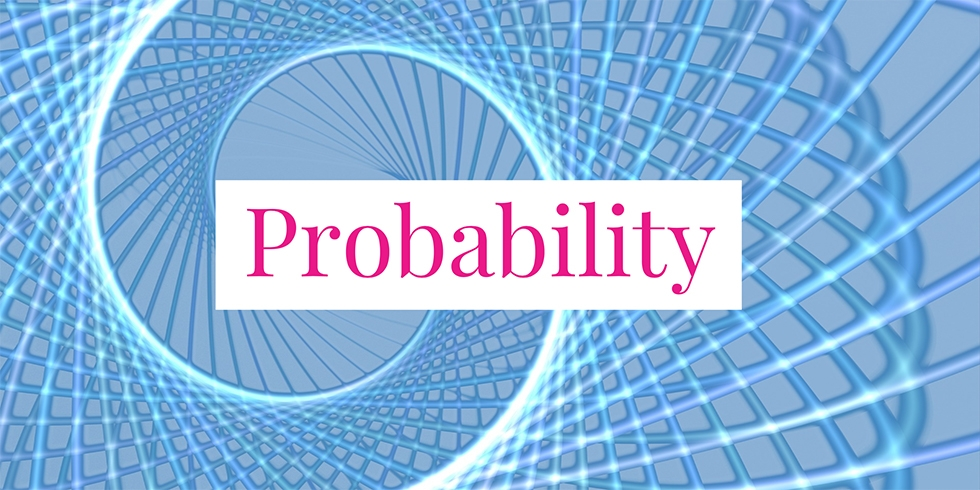# How to Prove Markov’s Inequality and Chebyshev’s Inequality## Problem 759

(a) Let $X$ be a random variable that takes only non-negative values. Prove that for any $a > 0$,
$P(X \geq a) \leq \frac{E[X]}{a}.$ This inequality is called Markov’s inequality.

(b) Let $X$ be a random variable with finite mean $\mu$ and variance $\sigma^2$. Prove that for any $a >0$,
$P\left(|X – \mu| \geq a \right) \leq \frac{\sigma^2}{a^2}.$ This inequality is called Chebyshev’s inequality.Add to solve later

## Solution.

We give two proofs of Markov’s inequality.

### First Proof of Markov’s Inequality

For the first proof, let us assume that $X$ is a discrete random variable. The case when $X$ is a continuous random variable is identical except summations are replaced by integrals. The mean $E[X]$ is by definition
\begin{align*}
E[X] &= \sum_{x, p(x)>0} x p(x).
\end{align*}
Here, each term $xp(x)$ is a non-negative number as $X$ is non-negative and $p(x)$ is a probability. Thus, omitting some terms reduces the sum.

Hence we have
\begin{align*}
E[X] &= \sum_{x} x p(x) \geq \sum_{x \geq a} x p(x).
\end{align*}
(We omitted those $x$ such that $x \lt a$.)

Now, since $x \geq a$, we have
$\sum_{x \geq a} x p(x) \geq \sum_{x \geq a} a p(x) = a \sum_{x \geq a} p(x).$ Note that
$\sum_{x \geq a} p(x) = P(X \geq a).$ It follows that we obtain
$E[X] \geq a P(X \geq a).$ Dividing this by $a>0$, we obtain the Markov’s inequality
$P(X \geq a) \leq \frac{E[X]}{a}.$

### Second Proof of Markov’s Inequality

Let us give an alternative proof. We define a new random variable $I$ by
\begin{align*}
I =
\begin{cases}
1 & \text{ if } X \geq a\\
0 & \text{ otherwise}.
\end{cases}
\end{align*}
(This is called an indicator variable for the event $X \geq a$.)

When $X \geq a$, we have $I = 1$. Thus,
$\frac{X}{a} \geq 1 = I.$ If, on the other hand, $X \lt a$, then as both $X$ and $a$ are non-negative, we have
$\frac{X}{a} \geq 0 = I.$ Therefore, in either case, we have the inequality
$\frac{X}{a} \geq I.$

This implies the inequality of their expected values
$E\left[\frac{X}{a}\right] \geq E[I].\tag{*}$ By linearity of expected value, we see that
$E\left[\frac{X}{a}\right] = \frac{E[X]}{a}.$ Also, we have
\begin{align*}
E[I] &= 0\cdot p(0) + 1\cdot p(1)\\
&= p(1)\\
&= P(X \geq a)
\end{align*}

It follows from (*) that
$\frac{E[X]}{a} \geq P(X \geq a).$ This completes the proof of Markov’s inequality.

### Proof of Chebyshev’s Inequality

The proof of Chebyshev’s inequality relies on Markov’s inequality.
Note that $|X – \mu| \geq a$ is equivalent to $(X-\mu)^2 \geq a^2$. Let us put
$Y = (X-\mu)^2.$ Then $Y$ is a non-negative random variable.

Applying Markov’s inequality with $Y$ and constant $a^2$ gives
\begin{align*}
P(Y \geq a^2) \leq \frac{E[Y]}{a^2}.
\end{align*}

Now, the definition of the variance of $X$ yields that
$E[Y]=E[(X-\mu)^2] = V[X] = \sigma^2.$

Combining these computations gives
\begin{align*}
P(|X-\mu| \geq a) &= P((X-\mu)^2 \geq a^2)\\[6pt] &= P(Y \geq a^2)\\[6pt] &\leq \frac{E[Y]}{a^2}\\[6pt] &= \frac{\sigma^2}{a^2},
\end{align*}
which concludes the proof of Chebyshev’s inequality.Add to solve later

### More from my site

#### You may also like...

This site uses Akismet to reduce spam. Learn how your comment data is processed.

###### More in Probability##### How to Use the Z-table to Compute Probabilities of Non-Standard Normal Distributions

Let $X\sim \mathcal{N}(\mu, \sigma)$ be a normal random variable with parameter $\mu=6$ and $\sigma^2=4$. Find the following probabilities using the...

Close